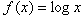Introduction

A transformation of a function shifts the parent function to another location on the coordinate plane. For this lesson we will focus on two types of transformations: translations and reflections. A translation shifts the graph left or right, or up or down. A reflection flips the graph across a line, such as the x or y-axis. For this lesson we will use the parent functions listed below:

 Linear: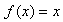Power: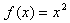or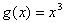Absolute Value: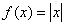Square Root: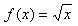Rational: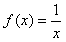or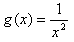Exponential: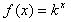Logarithmic: